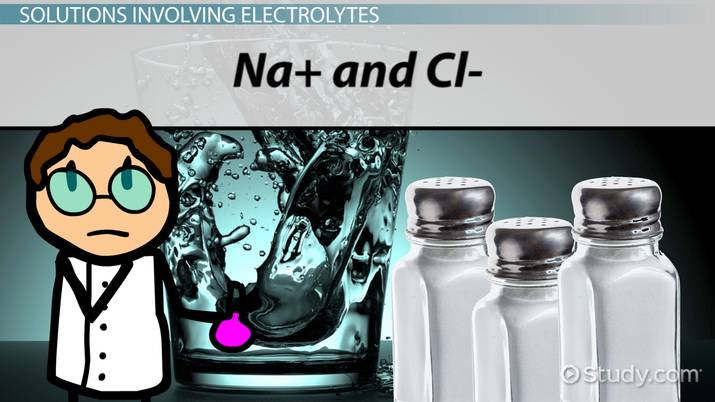# Calculating Boiling-Point Elevation of a Solution

Lesson Transcript
Instructor: Elizabeth (Nikki) Wyman

Nikki has a master's degree in teaching chemistry and has taught high school chemistry, biology and astronomy.

In this lesson, you will learn how to calculate the boiling point of a solution using a simple equation. Additionally, you will review the concepts of solutions, molality, and electrolytes.

## Background and Review

A pure substance has a specific boiling point at 1 atm. For water it's 100 degrees Celsius, for ethanol it's 78.4 degrees Celsius. What happens to the boiling point of a solution made of those two substances?

The boiling point of a solution varies depending on how much of each substance is present. There are two things that are always true, however: The boiling point of a solution is always higher than the pure substance, and it can be calculated relatively easily. Before we dive into equations, let's review a couple important ideas regarding solutions.

A solution is a homogenous mixture between two substances. The solute is a small amount of substance that is dissolved in another. The solvent is the liquid which dissolves the solute, and it occurs in a large quantity.

If you were making chocolate milk by adding two ounces of chocolate syrup to eight ounces of milk, the syrup is the solute and the milk is the solvent.

One of the most important properties of a solution is the ratio of solute to solvent, also known as the concentration. The letter 'm' represents molality (m), which is a means of expressing concentration that compares moles of solute to kilograms of solvent. The equation for molality is: m = mol solute/kg solvent.An error occurred trying to load this video.

Try refreshing the page, or contact customer support.

Coming up next: Acids and Bases

### You're on a roll. Keep up the good work!

Replay
Your next lesson will play in 10 seconds
• 0:00 Background and Review
• 1:29 The Boiling Point…
• 2:37 Boiling Point…
• 5:05 Solutions Involving…
• 6:49 Lesson Summary
Save Save

Want to watch this again later?

Timeline
Autoplay
Autoplay
Speed Speed

## The Boiling-Point Elevation Equation

The rather simple equation for determining boiling point of a solution: delta T = mKb.

Delta T refers to the boiling-point elevation, or how much greater the solution's boiling point is than that of the pure solvent. The units are degrees Celsius.

Kb is the molal boiling-point elevation constant. The value of Kb depends on the type of solvent present and nothing else. Since it's a constant, its value is always the same; you look up Kb for the solvent involved in a solution and plug the number in. It has the units degrees Celsius kg/mol. Here is a table of some common solvents, their normal boiling points and molal boiling-point elevation constants.

Solvent Boiling point Kb
Water 100 degrees C 0.51
Benzene 80.1 degrees C 2.53
Diethyl ether 34.5 degrees C 2.02

Lastly, m refers to molality. The units are mol/kg. Molality is an important concept in determining the boiling point of a solution. This is because the amount of solute present is what determines the boiling point. The identity of the solvent is not important.

## Boiling-Point Elevation Example

Let's imagine we need to determine the boiling point of a solution made of 36 grams of glucose dissolved in 300 grams of water. Let's list all the information we know about this problem, then solve it.

• Glucose is our solute and water is the solvent.
• Kb for water is 0.51 degrees Celsius kg/mol.
• The molar mass of glucose is 180 g/mol.
• The boiling point of water is 100 degrees Celsius.
• The equation for boiling-point elevation is delta T = mKb.

Let's study the equation for a second. We're solving for delta T and we already have Kb. All we need to do is determine molality and plug it into the equation.

molality = moles solute/kg solvent

To find the moles solute we divide 36 grams of glucose by the molar mass of glucose, or 180 g/mol. This is equal to 0.20 mol of glucose.

To find the kg of solvent, we just need to convert our 300 grams of water into kilograms. Since there are 1000 grams in a kilogram, we can find our kilograms of solvent by dividing 300 grams of water by 1000 grams to get 0.3000 kilograms of water.

Now, we can plug in our mol of solute and or kg of solvent into our molality equation:

molality = 0.20 mol glucose/0.3000 kg water = 0.67 mol/kg

Now that we have our molality, we can plug it into the boiling point elevation equation to find delta T, which equals 0.51 degrees Celsius kg/mol x 0.67 mol/kg.

Delta T = 0.34 degrees Celsius.

To unlock this lesson you must be a Study.com Member.

### Register to view this lesson

Are you a student or a teacher?